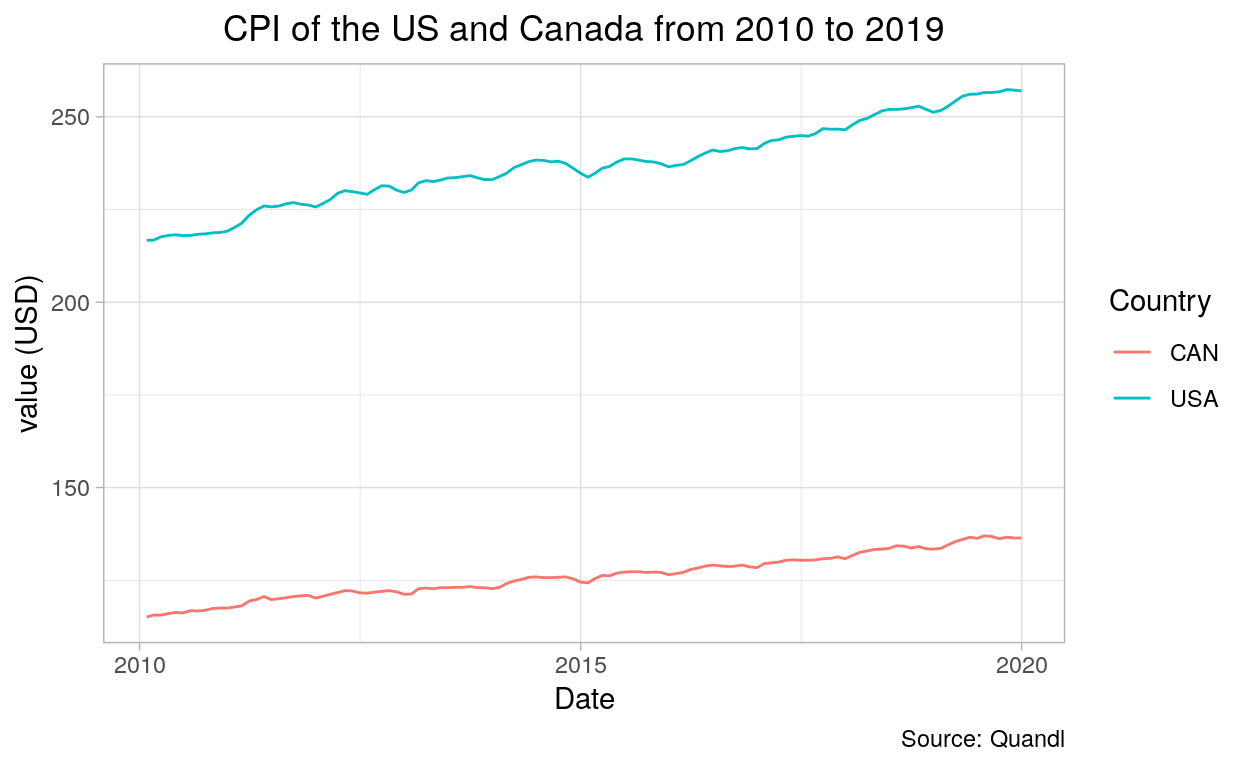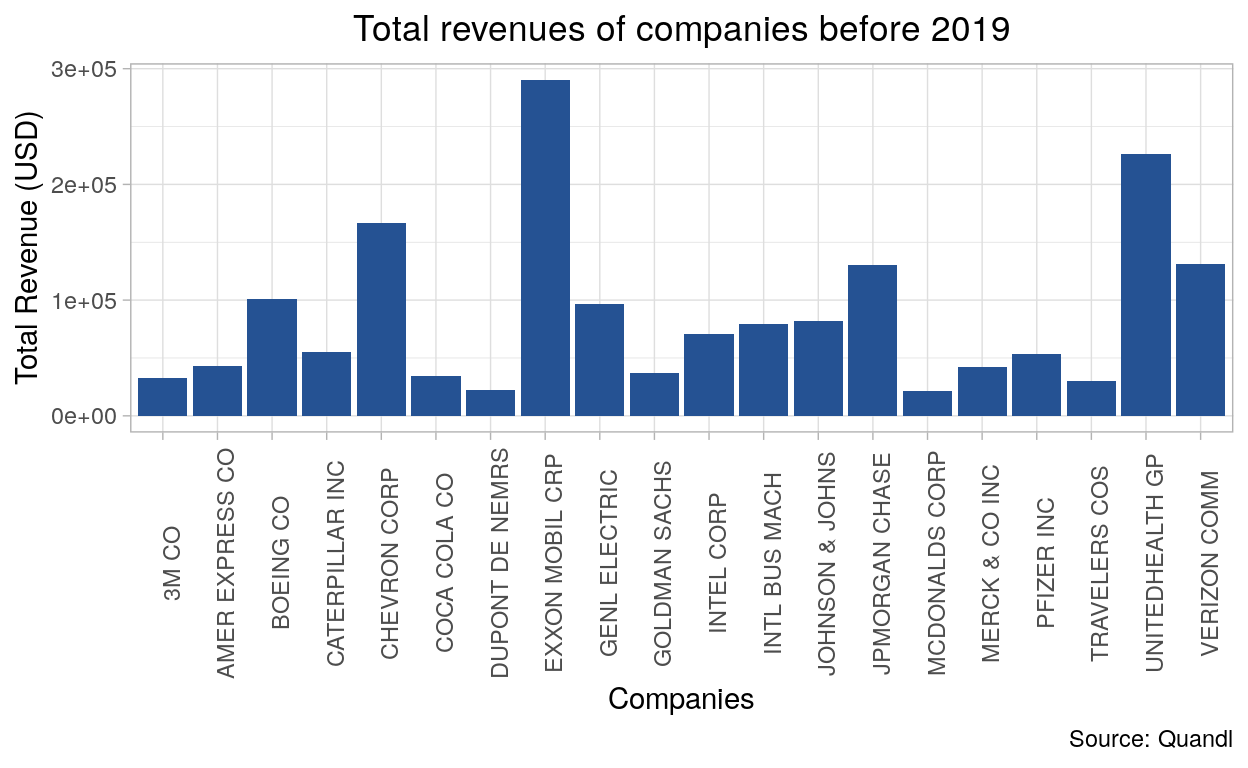# [API] Quandl: Application

Extract value from the world’s data

02-19-2020

This course will teach you how to use the Quandl package. Quandl allow you to collect financial and economic datasets from hundreds of publishers.

## Registering API key

``````# Load the fredR library
library(Quandl)

# Enter your API key in the function "Quandl.api_key"
Quandl.api_key("abcdefghijklmnopqrstuvwxyz123456")
``````

Before using the Quandl package, you have to (freely) register to the institution.

After confirming your email adress to validate the key, you will have to enter your API key in the function Quandl.api_key(). This will take the API Key given by Quandl and will allow the access to the database.

## Extracting data

### Quandl() function

``````# The Consumer Price Index of the US from 2010 to 2019

mydata_usa = Quandl("RATEINF/CPI_USA", start_date="2010-01-01", end_date="2019-12-31")

# The Consumer Price Index of Canada from 2010 to 2019

mydata_can = Quandl("RATEINF/CPI_CAN", start_date="2010-01-01", end_date="2019-12-31")
``````

### Quandl.datatable() function

``````# The total revenues of companies before 2019

mydatafun = Quandl.datatable("ZACKS/FC", per_type="A", per_end_date="2018-12-31", qopts.columns=c("m_ticker", "comp_name","currency_code", "tot_revnu", "per_type", "per_end_date"))
``````

## Visualizing data

The Consumer Price Index (line chart)

``````# Data Wrangling : add a new column

library(dplyr) # load the "dplyr" library

mydata_usa\$Country <- "USA" # add column "Country" and each variable in the column will be named USA

mydata_can\$Country <- "CAN" # add column "Country" and each variable in the column will be named CAN

df_new <- bind_rows(mydata_can, mydata_usa) # merge the 2 data frames together using the "bind_rows" function

library(ggplot2)

ggplot(data = df_new, aes(x = Date, y = Value, color = Country)) +
geom_line() +
theme_light() +
theme(plot.title = element_text(hjust = 0.5)) +
labs(title = "CPI of the US and Canada from 2010 to 2019",
x = "Date",
y = "value (USD)",
colour = "Country",
caption = "Source: Quandl")
``````Total revenues of companies before 2019 (bar chart)

``````library(ggplot2)

ggplot(data = mydatafun, aes(x = comp_name, y = tot_revnu)) +
geom_col(fill = "#255293") +
theme_light() +
theme(plot.title = element_text(hjust = 0.5)) +
labs(title = "Total revenues of companies before 2019",
x = "Companies",
y = "Total Revenue (USD)",
caption = "Source: Quandl") +
theme(axis.text.x = element_text(margin = margin(t = .1, unit = "cm"), angle = 90)) +
theme(legend.position = "none")
``````## Another example

``````# Load the following libraries
library(Quandl)
library(dplyr)
library(spdep)
library(maptools)
library(leaflet)
library(maps)
library(rgdal)
``````
``````# Enter your API key in the function "Quandl.api_key"
Quandl.api_key("abcdefghijklmnopqrstuvwxyz123456")
``````
``````# Extract your data from the Quandl package
JobUSA = Quandl.datatable("JL/JD2")

# Data Wrangling
names(JobUSA)[names(JobUSA) == "location_name"] <- "location" #rename column
names(JobUSA)[names(JobUSA) == "job_title"] <- "job" #rename column
names(JobUSA)[names(JobUSA) == "organization_country"] <- "country" #rename column
names(JobUSA)[names(JobUSA) == "region_label"] <- "NAME" #rename column
names(JobUSA)[names(JobUSA) == "organization_city"] <- "city" #rename column

JobUSA\$country <- gsub("US", "USA", JobUSA\$country) #change the term US for USA in the "country" column

JobUSAsub<- dplyr::select(JobUSA, NAME, posting_count) # select only relevant data in your data frame

JobUSAagg <- aggregate(posting_count ~ NAME, data = JobUSAsub, sum) # use the aggregate function to sum up entries in the "NAME" column that are the same

``````
``````OGR data source with driver: ESRI Shapefile
Source: "/home/marinel/portfolio/warin/_posts/api-quandl-application/cb_2018_us_state_500k.shp", layer: "cb_2018_us_state_500k"
with 56 features
It has 9 fields
Integer64 fields read as strings:  ALAND AWATER ``````
``````# Use "Left join" function to combine your two data frames

USmap@data <- left_join(USmap@data, JobUSAagg, by = "NAME")

# Create labels

USmap@data\$NAME <- as.character(USmap@data\$NAME)

USmap@data\$posting_count <- as.numeric(USmap@data\$posting_count)

labels <- sprintf("<strong>%s</strong><br/>%g", USmap@data\$NAME, USmap@data\$posting_count) %>% lapply(htmltools::HTML)

# Determinate the intervalls that will be shown in the map legend
bins <- c(0, 5, 10, 15, 20, 30, 40, 50, 60, 70)

# Choose a color scheme for your map

colors <- colorBin("Blues", domain = USmap@data\$posting_count, bins = bins)

# Plot the data using leaflet

leaflet(USmap) %>%
setView(lng = -95.712891, lat = 37.090240, zoom = 4) %>%
addLegend(pal = colors, values = USmap@data\$posting_count, opacity = 0.7, title = NULL, position = "bottomleft") %>%